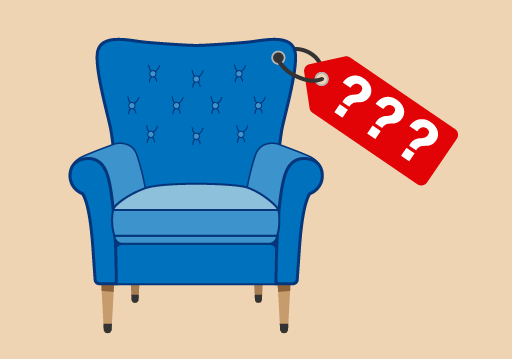Science, Maths & Technology

### Become an OU studentEveryday maths 1

Start this free course now. Just create an account and sign in. Enrol and complete the course for a free statement of participation or digital badge if available.

# 2.1 Estimating answers to calculations

Throughout this course you will be asked to estimate or approximate an answer in a scenario. If you do not use rounding to provide an answer to this question your answer will be incorrect.

Try the following activity using rounding throughout. Pay particular attention to the language used.

## Activity 7: Rounding

1. The population of a city is 6,439,800. Round this number to the nearest million.
2. Tickets to a concert cost £6 each. 6,987 tickets have been sold. Approximately how much money has been collected?
3. 412 students passed their Maths GCSE this year at Longfield High School. 395 passed last year. Approximately how many students passed GCSE Maths over the last two years?
4. Four armchairs cost £595. What is the approximate cost of one armchair?Figure 9 How much for one armchair?
5. A box contains 18 pencils. A company orders 50 boxes. Approximately how many pencils is that?

1. The population rounds to 6,000,000 (six million). This is because 6,439,800 is nearer to 6 million than 7 million.
2. 6,987 rounded to the nearest 1,000 is 7,000. If each ticket costs £6, the approximate total amount of money collected is:
• £6 × 7,000 = £42,000
3. 412 to the nearest hundred is 400. 395 to the nearest hundred is also 400. So the total approximate number of students passing GCSE Maths is:
• 400 + 400 = 800 students
4. £595 to the nearest hundred is £600. So the approximate cost of one armchair is:
• £600 ÷ 4 = £150
5. 18 rounded to the nearest 10 is 20. So the approximate total number of pencils is:

• 20 × 50 = 1,000 pencils

Note: 50 × 20 = 50 × 2 × 10 = 100 × 10 = 1,000.

## Summary

So far you have worked with negative numbers, whole numbers, estimation and multiples. All of the practised skills will help you with everyday tasks such as shopping, working with a budget and reading temperatures. The objectives that you have covered are:

• the meaning of a positive and negative number
• how to carry out calculations with whole numbers
• how an approximate answer can help to check an exact answer
• multiples and square numbers.

Later in this course you will be looking at inverse calculations. This means reversing all operations to check that your answer is correct.